The size of the standard paper DinA

The paper we usually use has a standar size. In lots of countries in the world (but not in North America) we use paper size standars based in ISO 216 and we use world like DIN A0, DIN A1, DIN A2, DIN A3, DIN A4 an so on.

The base DIN A0 size of paper is defined to have an area of one square meter, and successive paper sizes in the series A1, A2, A3, A4, and so forth, are defined by halving the preceding paper size along the larger dimension. The objective is that this parts again have the same aspect ratio.

We can calculate this aspect ratio: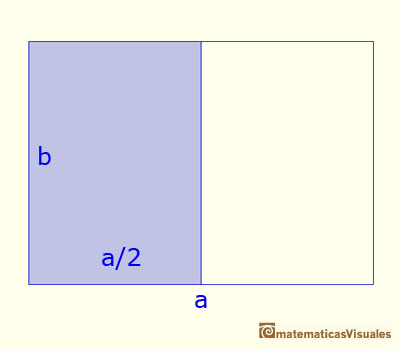The aspect ratio verifies (these two rectangles are similar):

Then

Or

Then the larger side is equal to the diagonal of a square of size the shorter side: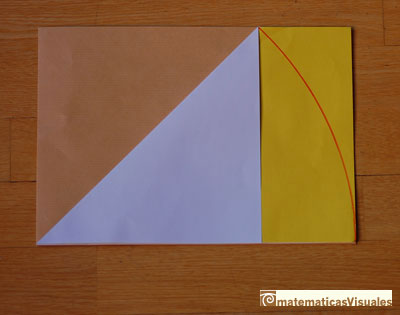DIN A0 size has one square meter. We can calculate his dimensions (rounded to milimeters)

In a photocopier, when we want to reduce from A3 to A4 the display shows a ratio of 71%. ¿Why?I have used this proportion in the animation about the sum of the geometric series of ratio 1/2.

The doors of this piece of furniture are in the same proportion. It has been designed and made by Roberto Cardil using pine and oak wood. You can see another furniture with the golden spiral.This proportion is different than the golden proportion.

Remember that the diagonal of a square is: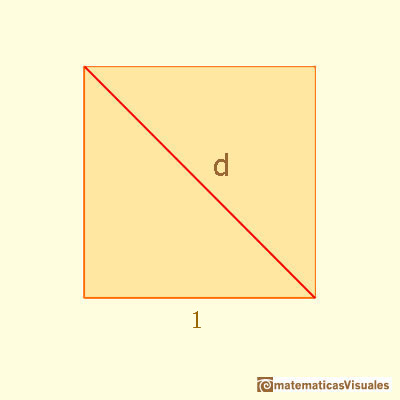Then we can find our rectangle as a section of a cube:One diagonal of this rectangle: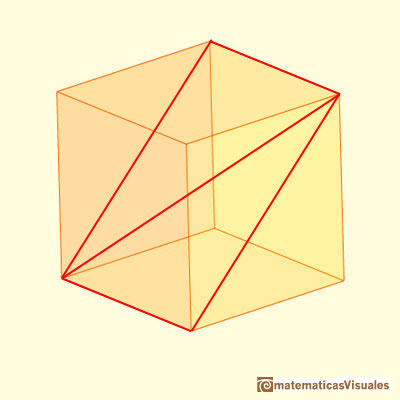You can calculate D as a basic application of the Pythagorean Theorem:If we consider the two diagonals of this section, the point of intersection is the center of the cube:Now we are going to study the angles between the two diagonals (we need some basic knowledge about trigonometry):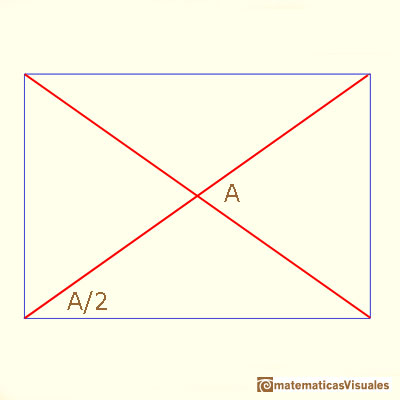Angle C is easy to calculate: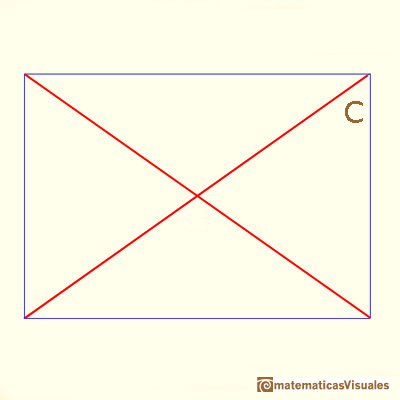AlsoWe are going to meet these two angles when we study the chamfered cube and the rhombic dodecahedron because our rectangle is related with these polyhedra.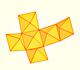A chain of six pyramids can be turned inwards to form a cube or turned outwards, placed over another cube to form the rhombic dodecahedron.You can chamfer a cube and then you get a polyhedron similar (but not equal) to a truncated octahedron. You can get also a rhombic dodecahedron.

Another approach to find angle A: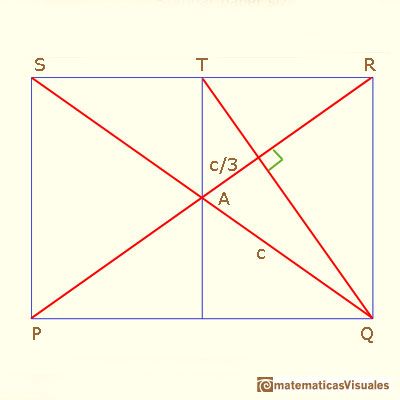Can you explain it?

First, lines PR and QT are perpendicular because if we rotate 90 degrees counterclockwise the rectangle ....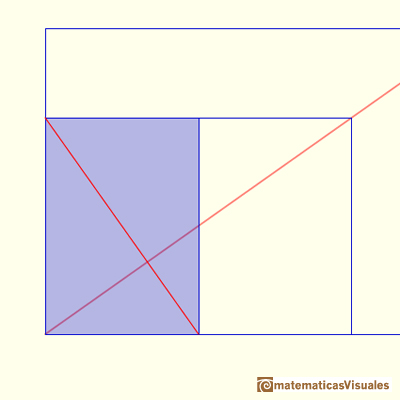Second, lines PR and QT are two medians of triangle QRS, then the centroid divide ...

Now you can write cosA ...You can chamfer a cube and then you get a polyhedron similar (but not equal) to a truncated octahedron. You can get also a rhombic dodecahedron.The geometric series of ratio 1/2 is convergent. We can represent this series using a rectangle and cut it in half successively. Here we use a rectangle such us all rectangles are similar.A golden rectangle is made of an square and another golden rectangle.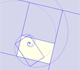A golden rectangle is made of an square an another golden rectangle. These rectangles are related through an dilative rotation.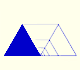One intuitive example of how to sum a geometric series. A geometric series of ratio less than 1 is convergent.You can build a Rhombic Dodecahedron adding six pyramids to a cube. This fact has several interesting consequences.Humankind has always been fascinated by how bees build their honeycombs. Kepler related honeycombs with a polyhedron called Rhombic Dodecahedron.We want to close a hexagonal prism as bees do, using three rhombi. Then, which is the shape of these three rhombi that closes the prism with the minimum surface area?.Adding six pyramids to a cube you can build new polyhedra with twenty four triangular faces. For specific pyramids you get a Rhombic Dodecahedron that has twelve rhombic faces.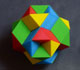Tetraxis is a wonderful puzzle designed by Jane and John Kostick. We study some properties of this puzzle and its relations with the rhombic dodecahedron. We can build this puzzle using cardboard and magnets or using a 3D printer.Starting with a Rhombicubotahedron we can add pyramids over each face. The we get a beautiful polyhedron that it is like a star.Material for a session about polyhedra (Zaragoza, 9th May 2014). Simple techniques to build polyhedra like the tetrahedron, octahedron, the cuboctahedron and the rhombic dodecahedron. We can build a box that is a rhombic dodecahedron.A Cube can be inscribed in a Dodecahedron. A Dodecahedron can be seen as a cube with six 'roofs'. You can fold a dodecahedron into a cube.Leonardo da Vinci made several drawings of polyhedra for Luca Pacioli's book 'De divina proportione'. Here we can see an adaptation of the cuboctahedron.A cuboctahedron is an Archimedean solid. It can be seen as made by cutting off the corners of a cube.A cuboctahedron is an Archimedean solid. It can be seen as made by cutting off the corners of an octahedron.